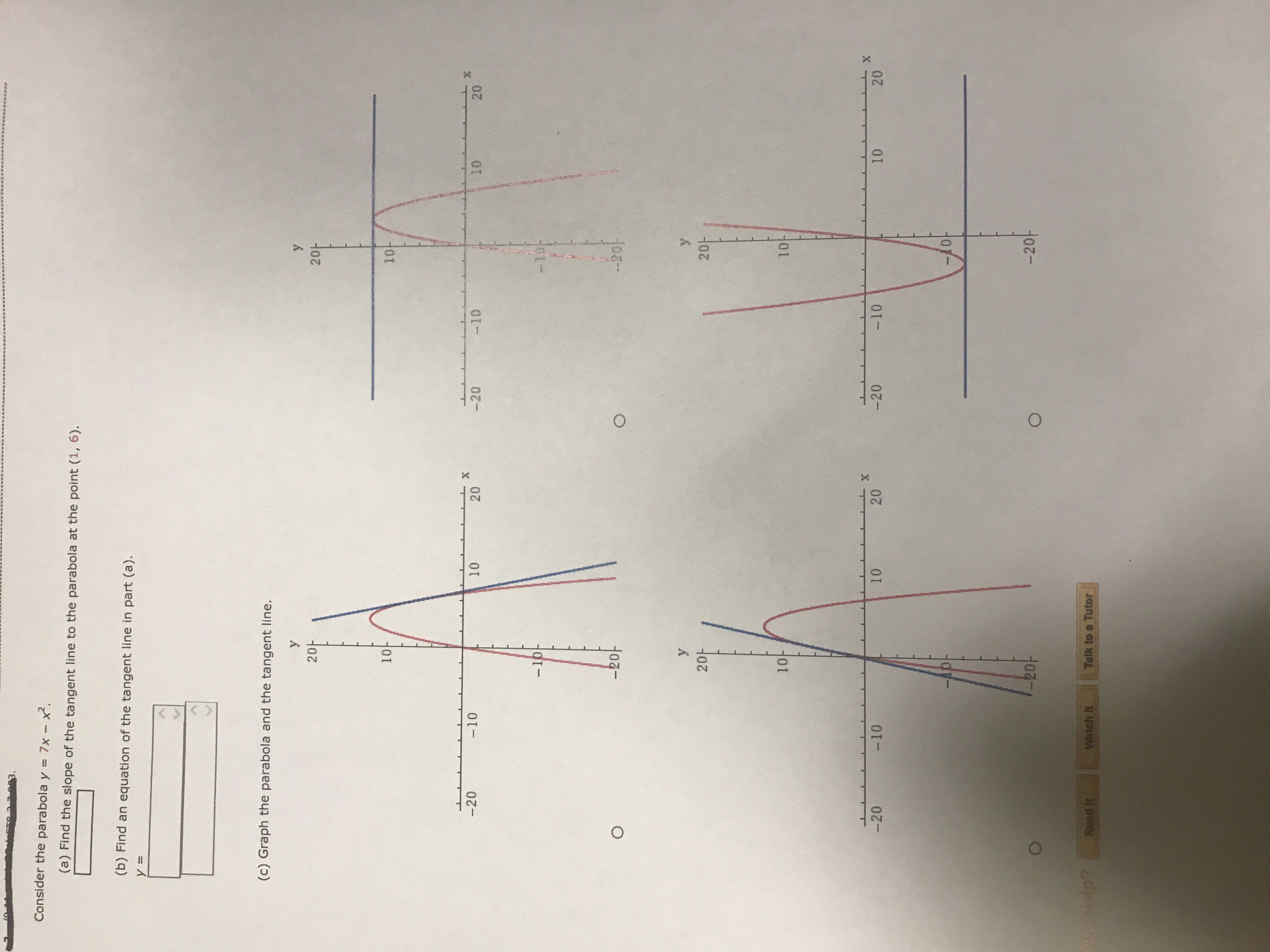Consider the parabola y7x-x.Find the slope of the tangent line to the parabola at the point (1, 6).(b) Find an equation of the tangent line in part (a)(c) Graph the parabola and the tangent line.2020마1010201020-20 10201002010101010201020-20-20-10-20Talk to Tutor

Questionhelp_outlineImage TranscriptioncloseConsider the parabola y 7x-x. Find the slope of the tangent line to the parabola at the point (1, 6). (b) Find an equation of the tangent line in part (a) (c) Graph the parabola and the tangent line. 20 20 마 10 10 20 10 20 -20 10 20 10 0 20 10 10 10 10 20 10 20 -20 -20 -10 -20 Talk to Tutor fullscreen
Step 1

Given equation of parabola y = 7x-x2

The slope of the tangent line at (x1, y1) is derivative of the given equation  y with respect to x at that given point.

Derivative of given equation with respect to x is shown below.

After substituting x = 1 from point (1,6) we get slope of tangent to parabola at (1,6) is 5

Step 2

The point slope form of a straight line with slope m and point (x1, y1) is shown below. Substituting the slope ...

Want to see the full answer?

See Solution

Want to see this answer and more?

Our solutions are written by experts, many with advanced degrees, and available 24/7

See Solution
Tagged in

Derivative